# Arrays and Strings and HashMap(?)

ArrayList -> An array that resizes itself when still needed while still providing O(1) access. A typical implementation is that when the array is full, the array doubles in size. Each doubling takes O(n) time, but happen rarely that its armortized insertion time is O(1).

StringBuilder -> On each concatenation, a new copy of the string is created, and the two strings are copied over, character by character.

Array -> 数组

Contiguous subarray -> 直数组

Element -> 元素

Sum -> 合, eg, 它们的合是。。。 Their sum is

# Common Techniques

## Sliding Window Approach

A window is formed over some part of the data and this window can slide over the data to capture different portions of it. When a window slides, only two elements change. The oldest one drops off and the newest one comes into the frame.

In the brute force approach, the inefficient part is that for any two consecutive subarrays of size k, the overlapping part (which contains k-1 elements) is evaluated twice. By using the sliding window approach, we can get rid of that and calculate the sum in O(1) using the sum of previous consecutive subarray.

You can also implement the sliding window approach with two pointers left & right as the following code.

The time complexity is then linear O(n) as we only need one loop.

`left = 0right = 0while right < n:    window.add(s[right])    right += 1        while (condition):        window.remove(s[left])        left += 1`

## Creating a character array to keep track of the occurrences of each character

`int[] counter(String word) {        int[] count = new int;        for (char c : word.toCharArray()) count[c - 'a']++;        return count;    }`

## Counting inversions

Use Merge sort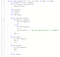Not sure why this solution has time exceeded error for the last few test cases on hacker rank.

## 315. Count of Smaller Numbers After Self

You are given an integer array nums and you have to return a new counts array. The counts array has the property where `counts[i]` is the number of smaller elements to the right of `nums[i]`.

Example 1:

`Input: nums = [5,2,6,1]Output: [2,1,1,0]Explanation:To the right of 5 there are 2 smaller elements (2 and 1).To the right of 2 there is only 1 smaller element (1).To the right of 6 there is 1 smaller element (1).To the right of 1 there is 0 smaller element.`

The optimal solution is to use MergeSort method to count.

We make use of 2 additional variables to help us. The first is int count[] which stores the result. The second is rightCount inside the merge method to help us in tracking number of right elements that is smaller than the current left element we are iterating.

Note that in merge, the items in the positions from [start…mid] and [mid+1…end] are already sorted internally. We term these two sorted portions to be LEFT and RIGHT.

In this merging, we are iterating through the indexes array. The p1, p2…indexes are the indexes of the elements in the LEFT and RIGHT respectively. We compare the values at those indexes p1, p2.

Here, we need to track its’ indexes and add to the new array depending on the values of these indexes (Doing merge sort on the index array and the basis of comparison is their values). We want to track the indexes because we need to edit count[] array.

** TDLR: We are only modifying the values in the indexes array. nums array remains unchanged.

Each time we add an element from the left side, we set the count[i] to be rightCount.

rightCount contains the number of elements on the right that is smaller than this current element on the left.

# Leetcode

## 27. Remove Element

Given an array nums and a value val, remove all instances of that value in-place and return the new length.

Do not allocate extra space for another array, you must do this by modifying the input array in-place with O(1) extra memory.

The order of elements can be changed. It doesn’t matter what you leave beyond the new length.

Return the last index of the modified array.

Example 1:

`Input: nums = [3,2,2,3], val = 3Output: 2, nums = [2,2]Explanation: Your function should return length = 2, with the first two elements of nums being 2.It doesn't matter what you leave beyond the returned length. For example if you return 2 with nums = [2,2,3,3] or nums = [2,3,0,0], your answer will be accepted.`

Example 2:

`Input: nums = [0,1,2,2,3,0,4,2], val = 2Output: 5, nums = [0,1,4,0,3]Explanation: Your function should return length = 5, with the first five elements of nums containing 0, 1, 3, 0, and 4. Note that the order of those five elements can be arbitrary. It doesn't matter what values are set beyond the returned length.`

## Q1 Given an array of integers, return the highest sum of any k consecutive elements in the array.

Wanted to use sliding window…but didn’t come up with a solution. So in the end, decide to use dynamic programming.

To remember how to solve this question, think of the sub problem:

• If i have the max sum of k consecutive elements from 0…i-1, and i am currently at index i, how do i make use of the results from prior? To form dp[i], how do i make use of dp[i-1]
• The first way we might think of is to have the 1D array, dp[i-1] contain the max sum of K consecutive elements from 0…...i–1, then decide whether to add curr element arr[i] to it or not. However, we soon realise that the element arr[i-1] might not have been included in the sum of dp[i-1]. Only when the previous element arr[i-1] has been added to dp[i-1], we can add arr[i] into the dp[i], otherwise it is not consecutive.
• Hence, we need a way to make it 100% correct for us to decide whether to add current element to dp[i].
• We then change our definition of what dp[i-1] contains.
• One way is to set the condition that dp[i-1] will always contain the maximum sum of elements from position i-1 (Ensure that the prev element is always added to the sum) to somewhere ≥ 0. Hence, in each iteration we are safe to append arr[i] to the sum of dp[i]. Then we can make it such that in each iteration, we are only deciding whether we want to add current element a[i] to dp[i-1] or start afresh with a[i]. We then have to keep track of the maximum sum by having a variable.

## Q2 Given a string, find the length of the longest substring without repeating characters.

Approach 1: Naive brute force where you try every combination of substrings

Approach 2: Optimised brute force. For each starting index i, find the longest substring. O(n)*O(n)=O(n²)

Approach 3:

Can use HashMap

Hard question…i could not figure out how to do the hashmap method as suggested by huahua. Spent a good hour trying and failing…then I copied this solution below from youtube….my brain just isnt working…

currSubstring.substring(1); // Means only keep characters after the first.

# 340. Longest Substring with At Most K Distinct Characters

Given a string, find the length of the longest substring T that contains at most k distinct characters.

For example, Given s = `“eceba”` and k = 2,

T is “ece” which its length is 3.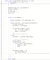Not super sure of the answer but it passes the two test cases i see from the other websites to obtain this premium leet code question.

## You may assume that each input would have exactly one solution, and you may not use the same element twice.

Example:

`Given nums = [2, 7, 11, 15], target = 9,Because nums + nums = 2 + 7 = 9,return [0, 1].`

# Map<Integer, Integer> map = new HashMap<Integer, Integer>();

new int[] {1,2,3,4};

Need to be clear of what values you are storing in the Map.

## Q3 Three Sum: Given an array `nums` of n integers, are there elements a, b, c in `nums` such that a + b + c = 0? Find all unique triplets in the array which gives the sum of zero.

Note:

The solution set must not contain duplicate triplets.

Example:

`Given array nums = [-1, 0, 1, 2, -1, -4],A solution set is:[  [-1, 0, 1],  [-1, -1, 2]]`

This solution does not pass the time-complexity..but i spent very long to come up with it so i shall keep it. It passes 311/313 test cases on leet code.

Found another much better solution in the discussion forum. This solution does not use TwoSum. Instead, it uses modified binary search.

## Q4 Longest Common subsequence Leetcode 1143

Given two strings `text1` and `text2`, return the length of their longest common subsequence.

A subsequence of a string is a new string generated from the original string with some characters(can be none) deleted without changing the relative order of the remaining characters. (eg, “ace” is a subsequence of “abcde” while “aec” is not). A common subsequence of two strings is a subsequence that is common to both strings.

If there is no common subsequence, return 0.

Example 1:

`Input: text1 = "abcde", text2 = "ace" Output: 3  Explanation: The longest common subsequence is "ace" and its length is 3.`

Example 2:

`Input: text1 = "abc", text2 = "abc"Output: 3Explanation: The longest common subsequence is "abc" and its length is 3.`

Example 3:

`Input: text1 = "abc", text2 = "def"Output: 0Explanation: There is no such common subsequence, so the result is 0.`

The idea is to have 2D matrix where each index dp[i][j] represents the longest substring of the two strings: string1[0…..i] and string2[0…..j].

If you need to print out the string:

# Cracking the coding interview

## (1) Is Unique: Implement an algorithm to determine if a string has all unique characters. Cannot use additional data structures?

Approach 1: Brute Force 2 For Loop

Approach 2: Sorting O(nlogn)

Approach 3: Use of extra data structures O(n) -> Can use HashMap

Approach 4: Without extra data structure

## (2) Does string2 contains permutation of string1? (Leet code)

found this hard...spent 2 hours and in the end just gave up by looking at the solution.

Given two strings s1 and s2, write a function to return true if s2 contains the permutation of s1. In other words, one of the first string’s permutations is the substring of the second string.

Example 1:

`Input: s1 = "ab" s2 = "eidbaooo"Output: TrueExplanation: s2 contains one permutation of s1 ("ba").`

Example 2:

`Input: s1= "ab" s2 = "eidboaoo"Output: False`
• Use sliding window approach here. The size of the window is length of string 1.
• Each time we iterate through String 2, we check if the count of characters for both are the same.
• Before line 11 is reached, the s1map and s2map will contain the mapping of the characters in both string 1 and a subset of string 2. Substring of length string 1 of string 2.
• Then we will have 2 maps called s1map and s2map.
• Afterwards, we immediately check whether they have the same values via matches function.
• Then, if it doesn’t match, we will go on to change the map of S2 by checking the next character of string2. For eg, if string1 is of length X and string2 is of length Y, then the next char to be explored will be at position (Y-X-1). This is line 14.
• In line 15, we will attempt to remove the first character of string2 at position 0.
• So everytime we check for similarity in the match function, we are keeping the size of the window to the length of string 1.

## (3) Leet Code 49 Group Anagrams

Given an array of strings, group anagrams together.

Example:

`Input: ["eat", "tea", "tan", "ate", "nat", "bat"],Output:[  ["ate","eat","tea"],  ["nat","tan"],  ["bat"]]`

First things to think of -> Use of Hash map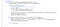My initial solution..but this solution only passes 100 out of 101 test cases. Meaning that to identify strings, we cannot use the multiplications of the characters ascii code.

One correct solution is to set the key of the HashMap to be the sorted string of each anagram. For eg, if we have “cat”, “tac”, “cta”, the key of this group would be “act” which is the sorted version of those strings.

`char[] ca = eachString.toCharArray(); //Convert to array of charactersArrays.sort(ca) //Sort it;String key = String.valueOf(ca); //Convert back to string format`

Note that when we want to do integer, we do Integer.parseInt();

## (5) Leet Code Rotate Image (Matrix)

You are given an n x n 2D matrix representing an image.

Rotate the image by 90 degrees (clockwise).

Note:

You have to rotate the image in-place, which means you have to modify the input 2D matrix directly. DO NOT allocate another 2D matrix and do the rotation.

Example 1:

`Given input matrix = [  [1,2,3],  [4,5,6],  [7,8,9]],rotate the input matrix in-place such that it becomes:[  [7,4,1],  [8,5,2],  [9,6,3]]`

Example 2:

`Given input matrix =[  [ 5, 1, 9,11],  [ 2, 4, 8,10],  [13, 3, 6, 7],  [15,14,12,16]], rotate the input matrix in-place such that it becomes:[  [15,13, 2, 5],  [14, 3, 4, 1],  [12, 6, 8, 9],  [16, 7,10,11]]`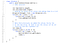Correction: O(N²) Solution where N is length of a side of the matrix

# (6) Leet Code 274 H-Index

Given an array of citations (each citation is a non-negative integer) of a researcher, write a function to compute the researcher’s h-index.

According to the definition of h-index on Wikipedia: “A scientist has index h if h of his/her N papers have at least h citations each, and the other N − h papers have no more than h citations each.”

Example:

`Input: citations = [3,0,6,1,5]Output: 3 Explanation: [3,0,6,1,5] means the researcher has 5 papers in total and each of them had received 3, 0, 6, 1, 5 citations respectively. Since the researcher has 3 papers with at least 3 citations each and the remaining two with no more than 3 citations each, her h-index is 3.`

Note: If there are several possible values for h, the maximum one is taken as the h-index.

If the number of citation papers we have seen (excluding the current one) is bigger or equal to the current h value, then return the number of citation papers seen.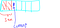The array here is sorted in descending order — diff from my code

See X papers, all must be at least h value — current. Compare number of X paper with h value. We want number of papers X to be ≥ h.

## 7) Leet Code 150. Evaluate Reverse Polish Notation

Reverse polish notation -> Postfix notation in which operators follow their operands.

Polish notation -> Operators precede their operands.

The reverse Polish scheme was proposed in 1954 by Arthur Burks, Don Warren, and Jesse Wright and was independently reinvented by Friedrich L. Bauer and Edsger W. Dijkstra in the early 1960s to reduce computer memory access and utilize the stack to evaluate expressions

Evaluate the value of an arithmetic expression in Reverse Polish Notation.

Valid operators are `+`, `-`, `*`, `/`. Each operand may be an integer or another expression.

Note:

• Division between two integers should truncate toward zero.
• The given RPN expression is always valid. That means the expression would always evaluate to a result and there won’t be any divide by zero operation.

Example 1:

`Input: ["2", "1", "+", "3", "*"]Output: 9Explanation: ((2 + 1) * 3) = 9`

Example 2:

`Input: ["4", "13", "5", "/", "+"]Output: 6Explanation: (4 + (13 / 5)) = 6`

When the current element is not a number, we will pop out 2 elements from the stack and perform the operation. When the current element is a number, we just append it to the stack.

Another way to check if the current item is an integer, we can convert it to a character.

`Character.isDigit(characterDigit)`

Note that item==“*” or “/” or “+” or “-” does not work. Need to use contain.

## 8) Leet Code 14 Longest Common Prefix

Write a function to find the longest common prefix string among an array of strings.

If there is no common prefix, return an empty string `""`.

Example 1:

`Input: ["flower","flow","flight"]Output: "fl"`

Example 2:

`Input: ["dog","racecar","car"]Output: ""Explanation: There is no common prefix among the input strings.`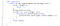Has to be index of -> because we want their first few characters to match
1. Set prefix to be the first string in the strs array
2. As we iterate through the other elements of the string array, we make the prefix smaller and smaller if it does not exist in the first few parts of the string. We remove the last character.

## 9) Leetcode 179 form the largest number

Given a list of non negative integers, arrange them such that they form the largest number.

Example 1:

`Input: [10,2]Output: "210"`

Using comparator:

It returns a positive value if obj1 is greater than obj2. Otherwise, a negative value is returned. By overriding compare( ), you can alter the way that objects are ordered.

• NumComparator will arrange a in front of b => (a,b) if we return a positive value.
• If we want b to be arranged in front of a => (b,a) We need a negative value to be returned. When do we want this arrangement, (b,a)? When (b+a) > (a+b).
• String compareTo: If first string is lexicographically greater than second string, it returns positive number.
• Hence, we want to make sure that we compare (b+a).compareTo(a+b) which corresponds to the code above.
• order2.compareTo( order1); Returns negative when order1 is smaller. Hence, when order1 is smaller, it means a+b < b+a. This means we dont want a to be put in front of b and we can directly return.

## 10. ZigZag Conversion

The string `"PAYPALISHIRING"` is written in a zigzag pattern on a given number of rows like this: (you may want to display this pattern in a fixed font for better legibility)

`P   A   H   NA P L S I I GY   I   R`

And then read line by line: `"PAHNAPLSIIGYIR"`

Write the code that will take a string and make this conversion given a number of rows:

`string convert(string s, int numRows);`

Example 1:

`Input: s = "PAYPALISHIRING", numRows = 3Output: "PAHNAPLSIIGYIR"`

Example 2:

`Input: s = "PAYPALISHIRING", numRows = 4Output: "PINALSIGYAHRPI"Explanation:P     I    NA   L S  I GY A   H RP     I`
• Create an index that iterate from row 0…numRows, then back to 0 and repeat.

## 11. Leetcode 916 Word Subsets

We are given two arrays `A` and `B` of words. Each word is a string of lowercase letters.

Now, say that word `b` is a subset of word `a` if every letter in `b` occurs in `a`, including multiplicity. For example, `"wrr"` is a subset of `"warrior"`, but is not a subset of `"world"`.

Now say a word `a` from `A` is universal if for every `b` in `B`, `b` is a subset of `a`.

Return a list of all universal words in `A`. You can return the words in any order.

Example 1:

`Input: A = ["amazon","apple","facebook","google","leetcode"], B = ["e","o"]Output: ["facebook","google","leetcode"]`

## 12 Leetcode 376 Wriggle Subsequence

A sequence of numbers is called a wiggle sequence if the differences between successive numbers strictly alternate between positive and negative. The first difference (if one exists) may be either positive or negative. A sequence with fewer than two elements is trivially a wiggle sequence.

For example, `[1,7,4,9,2,5]` is a wiggle sequence because the differences `(6,-3,5,-7,3)` are alternately positive and negative. In contrast, `[1,4,7,2,5]` and `[1,7,4,5,5]` are not wiggle sequences, the first because its first two differences are positive and the second because its last difference is zero.

Given a sequence of integers, return the length of the longest subsequence that is a wiggle sequence. A subsequence is obtained by deleting some number of elements (eventually, also zero) from the original sequence, leaving the remaining elements in their original order.

## 13 Leetcode 324 Wiggle Sort II

Given an unsorted array `nums`, reorder it such that `nums < nums > nums < nums...`.

Example 1:

`Input: nums = [1, 5, 1, 1, 6, 4]Output: One possible answer is [1, 4, 1, 5, 1, 6].`

Example 2:

`Input: nums = [1, 3, 2, 2, 3, 1]Output: One possible answer is [2, 3, 1, 3, 1, 2].`

Solution #1

A pretty simple and straightforward solution is:

1. Sort the array (with an auxiliary array)
2. Copy the elements to the correct positions

It’s the code:

`class Solution {    public void wiggleSort(int[] nums) {        int[] val = Arrays.copyOf(nums, nums.length);        Arrays.sort(val);        int idx = val.length - 1;        for(int i = 1;i < nums.length;i += 2) nums[i] = val[idx--];        for(int i = 0;i < nums.length;i += 2) nums[i] = val[idx--];    }}`

Note that we don’t need to calculate all the concrete positions to be copied; we just need to go through the array by odd and even positions till exhausting the array.

The space complexity is O(N), and the time complexity is O(NlogN) + O(N) (sorting + filling) = O(NlogN).

Solution #2

To achieve space complexity O(1) and time complexity O(N), a popular approach is:

The idea is based on the fact that if we make sure that all even positioned (at index 0, 2, 4, ..) elements are greater than their adjacent odd elements.

We traverse an array once, visiting all even positions. Then, make sure that the numbers at even positions are greater than its left and right elements. If it is not bigger, then we swap them.

# Leet code 966 Vowel Spellchecker

Given a `wordlist`, we want to implement a spellchecker that converts a query word into a correct word.

For a given `query` word, the spell checker handles two categories of spelling mistakes:

Capitalization: If the query matches a word in the wordlist (case-insensitive), then the query word is returned with the same case as the case in the wordlist.

• Example: `wordlist = ["yellow"]`, `query = "YellOw"`: `correct = "yellow"`
• Example: `wordlist = ["Yellow"]`, `query = "yellow"`: `correct = "Yellow"`
• Example: `wordlist = ["yellow"]`, `query = "yellow"`: `correct = "yellow"`

Vowel Errors: If after replacing the vowels (‘a’, ‘e’, ‘i’, ‘o’, ‘u’) of the query word with any vowel individually, it matches a word in the wordlist (case-insensitive), then the query word is returned with the same case as the match in the wordlist.

• Example: `wordlist = ["YellOw"]`, `query = "yollow"`: `correct = "YellOw"`
• Example: `wordlist = ["YellOw"]`, `query = "yeellow"`: `correct = ""` (no match)
• Example: `wordlist = ["YellOw"]`, `query = "yllw"`: `correct = ""` (no match)

In addition, the spell checker operates under the following precedence rules:

• When the query exactly matches a word in the wordlist (case-sensitive), you should return the same word back.
• When the query matches a word up to capitalization, you should return the first such match in the wordlist.
• When the query matches a word up to vowel errors, you should return the first such match in the wordlist.
• If the query has no matches in the wordlist, you should return the empty string.

Given some `queries`, return a list of words `answer`, where `answer[i]` is the correct word for `query = queries[i]`.

Note that all cases are exclusive….Need to be clear of the question.

## Leet Code 1024 Video Stitching

You are given a series of video clips from a sporting event that lasted `T` seconds. These video clips can be overlapping with each other and have varied lengths.

Each video clip `clips[i]` is an interval: it starts at time `clips[i]` and ends at time `clips[i]`. We can cut these clips into segments freely: for example, a clip `[0, 7]` can be cut into segments `[0, 1] + [1, 3] + [3, 7]`.

Return the minimum number of clips needed so that we can cut the clips into segments that cover the entire sporting event (`[0, T]`). If the task is impossible, return `-1`.

Example 1:

`Input: clips = [[0,2],[4,6],[8,10],[1,9],[1,5],[5,9]], T = 10Output: 3Explanation: We take the clips [0,2], [8,10], [1,9]; a total of 3 clips.Then, we can reconstruct the sporting event as follows:We cut [1,9] into segments [1,2] + [2,8] + [8,9].Now we have segments [0,2] + [2,8] + [8,10] which cover the sporting event [0, 10].`

Example 2:

`Input: clips = [[0,1],[1,2]], T = 5Output: -1Explanation: We can't cover [0,5] with only [0,1] and [1,2].`

Example 3:

`Input: clips = [[0,1],[6,8],[0,2],[5,6],[0,4],[0,3],[6,7],[1,3],[4,7],[1,4],[2,5],[2,6],[3,4],[4,5],[5,7],[6,9]], T = 9Output: 3Explanation: We can take clips [0,4], [4,7], and [6,9].`

Example 4:

`Input: clips = [[0,4],[2,8]], T = 5Output: 2Explanation: Notice you can have extra video after the event ends.`

## Leet code 215. Kth Largest Element in an Array

Find the kth largest element in an unsorted array. Note that it is the kth largest element in the sorted order, not the kth distinct element.

Example 1:

`Input: [3,2,1,5,6,4] and k = 2Output: 5`

Example 2:

`Input: [3,2,3,1,2,4,5,5,6] and k = 4Output: 4`

The idea: Quick Select algorithm

The condition at the WHILE loop has to be while left ≤ right. If we remove equality, then we miss the below case where we only have 1 element …

## Leetcode 973. K Closest Points to Origin

We have a list of `points` on the plane. Find the `K` closest points to the origin `(0, 0)`.

(Here, the distance between two points on a plane is the Euclidean distance.)

You may return the answer in any order. The answer is guaranteed to be unique (except for the order that it is in.)

Example 1:

`Input: points = [[1,3],[-2,2]], K = 1Output: [[-2,2]]Explanation: The distance between (1, 3) and the origin is sqrt(10).The distance between (-2, 2) and the origin is sqrt(8).Since sqrt(8) < sqrt(10), (-2, 2) is closer to the origin.We only want the closest K = 1 points from the origin, so the answer is just [[-2,2]].`

Example 2:

`Input: points = [[3,3],[5,-1],[-2,4]], K = 2Output: [[3,3],[-2,4]](The answer [[-2,4],[3,3]] would also be accepted.)`

Leet Code Solution:

## Leetcode 43 Multiply Strings

Given two non-negative integers `num1` and `num2` represented as strings, return the product of `num1` and `num2`, also represented as a string.

Example 1:

`Input: num1 = "2", num2 = "3"Output: "6"`

Example 2:

`Input: num1 = "123", num2 = "456"Output: "56088"`

Note:

1. The length of both `num1` and `num2` is < 110.
2. Both `num1` and `num2` contain only digits `0-9`.
3. Both `num1` and `num2` do not contain any leading zero, except the number 0 itself.
4. You must not use any built-in BigInteger library or convert the inputs to integer directly.

## Leet code 780 Reaching Points

A move consists of taking a point `(x, y)` and transforming it to either `(x, x+y)` or `(x+y, y)`.

Given a starting point `(sx, sy)` and a target point `(tx, ty)`, return `True` if and only if a sequence of moves exists to transform the point `(sx, sy)` to `(tx, ty)`. Otherwise, return `False`.

`Examples:Input: sx = 1, sy = 1, tx = 3, ty = 5Output: TrueExplanation:One series of moves that transforms the starting point to the target is:(1, 1) -> (1, 2)(1, 2) -> (3, 2)(3, 2) -> (3, 5)Input: sx = 1, sy = 1, tx = 2, ty = 2Output: FalseInput: sx = 1, sy = 1, tx = 1, ty = 1Output: True`

First, we keep reducing tx and ty to values until one of the tx==sx or ty==sy. At this point, we know we are at a point located either horizontal from the source or vertical from the source.

So if it is located horizontally away from source, then ty==sy will be true. So we keep reducing tx value. We see if we can move from tx to sx with ty steps.

## Leetcode 300 Longest Increasing Subsequence

Given an unsorted array of integers, find the length of longest increasing subsequence.

Example:

`Input: [10,9,2,5,3,7,101,18]Output: 4 Explanation: The longest increasing subsequence is [2,3,7,101], therefore the length is 4.`

We need to have a variable max instead of returning dp[last index] because the last element might not be included in the longest sequence.

## Leet code 775. Global and Local Inversions

We have some permutation `A` of `[0, 1, ..., N - 1]`, where `N` is the length of `A`.

The number of (global) inversions is the number of `i < j` with `0 <= i < j < N` and `A[i] > A[j]`.

The number of local inversions is the number of `i` with `0 <= i < N` and `A[i] > A[i+1]`.

Return `true` if and only if the number of global inversions is equal to the number of local inversions.

• NOTE THAT: The values in the array are 0,1…n

Example 1:

`Input: A = [1,0,2]Output: trueExplanation: There is 1 global inversion, and 1 local inversion.`

Example 2:

`Input: A = [1,2,0]Output: falseExplanation: There are 2 global inversions, and 1 local inversion.`

## 1249. Minimum Remove to Make Valid Parentheses

Given a string s of `'('` , `')'` and lowercase English characters.

Your task is to remove the minimum number of parentheses ( `'('` or `')'`, in any positions ) so that the resulting parentheses string is valid and return any valid string.

Formally, a parentheses string is valid if and only if:

• It is the empty string, contains only lowercase characters, or
• It can be written as `AB` (`A` concatenated with `B`), where `A` and `B` are valid strings, or
• It can be written as `(A)`, where `A` is a valid string.

Example 1:

`Input: s = "lee(t(c)o)de)"Output: "lee(t(c)o)de"Explanation: "lee(t(co)de)" , "lee(t(c)ode)" would also be accepted.`

Example 2:

`Input: s = "a)b(c)d"Output: "ab(c)d"`

Example 3:

`Input: s = "))(("Output: ""Explanation: An empty string is also valid.`

Example 4:

`Input: s = "(a(b(c)d)"Output: "a(b(c)d)"`
• Use a stack to track the indices of the open brackets.
• Whenever you see a closing bracket, pop one out of the stack. If the stack is empty, it means we are dealing with an extra closing bracket. However, we do not immediately remove it. Instead, we do a lazy deletion by substituting it with a symbol #. This is because, later on, when we want to remove the open brackets, the indices is based on the original string. Hence, we want these indices to remain valid.
• At the end of going through all characters, if there are excess open brackets in the stack, we will then need to remove them.
• At the last part, we remove the closing brackets that were lazily removed previously by doing a replaceAll(“#”, “”).
• Note that the commented lines are the same as line 37. However, it is less efficient that line 37 as it does not pass the last test case on leet code.

## 301. Remove Invalid Parentheses **

Remove the minimum number of invalid parentheses in order to make the input string valid. Return all possible results.

Note: The input string may contain letters other than the parentheses `(` and `)`.

Example 1:

`Input: "()())()"Output: ["()()()", "(())()"]`

Example 2:

`Input: "(a)())()"Output: ["(a)()()", "(a())()"]`

Example 3:

`Input: ")("Output: [""]`

The idea is to make use of recursion to remove brackets from the string. As long as the current string is not valid, we try to remove one character at different positions from the string (For loop in line 35–39).

Then, once we have a valid string, we add to results, provided that we checked it is the minimum.

Line 29 might not be needed actually.

The seenBefore Hash Set is to help in the time complexity where we store all those strings arrangements that we have seen before. This helps make sure we do not repeat recursion calls on the same strings.

## 921. Minimum Add to Make Parentheses Valid **

Given a string `S` of `'('` and `')'` parentheses, we add the minimum number of parentheses ( `'('` or `')'`, and in any positions ) so that the resulting parentheses string is valid.

Formally, a parentheses string is valid if and only if:

• It is the empty string, or
• It can be written as `AB` (`A` concatenated with `B`), where `A` and `B` are valid strings, or
• It can be written as `(A)`, where `A` is a valid string.

Given a parentheses string, return the minimum number of parentheses we must add to make the resulting string valid.

Example 1:

`Input: "())"Output: 1`

Example 2:

`Input: "((("Output: 3`

Example 3:

`Input: "()"Output: 0`

Example 4:

`Input: "()))(("Output: 4`

Line 10: Found a match

Line 11: If the program enters here, it means there is not enough openBrack to match the current close bracket. Hence, need to add one more open ‘(’ bracket.

Line 17: toAddCloseBrack contains the number of unmatched ‘(’ brackets. Hence, this variable tells us the number of close ‘)’ brackets to add.

## 32. Longest Valid Parentheses

Pretty challenging

Given a string containing just the characters `'('` and `')'`, find the length of the longest valid (well-formed) parentheses substring.

Example 1:

`Input: s = "(()"Output: 2Explanation: The longest valid parentheses substring is "()".`

Example 2:

`Input: s = ")()())"Output: 4Explanation: The longest valid parentheses substring is "()()".`

Example 3:

`Input: s = ""Output: 0`

Leet Code Solution:

The ith element of dp represents the length of the longest valid substring ending at ith index.

Now, it’s obvious that the valid substrings must end with ‘)’. This further leads to the conclusion that the substrings ending with ‘(’ will always contain ‘0’ at their corresponding dp indices. Thus, we update the dp array only when ‘)’ is encountered.

i-dp[i-1] gives us the starting position of the previous valid string. We do a -1 to i-dp[i-1] to get the character before it.

1. If the character at i-1 is a ‘)’, then we check where is the starting index of the valid substring that ends with i-1? This is given by i-dp[i-1].
2. Then, we want to know if there is a ‘( in front of this valid substring. So we check i-dp[i-1]-1 and check if it is == ‘(’.
3. If yes, then we need to check the value of dp at the position i-dp[i-1]-2 (olive bracket). If the value dp[i-dp[i-1–2] was previously ≥ 2, then we can add this value (olive)+ 2 (pink and lime green current matching brackets) + most recent valid substring (blue brackets) given by dp[i-1].

## Basic Calculator I

Implement a basic calculator to evaluate a simple expression string.

The expression string may contain open `(` and closing parentheses `)`, the plus `+` or minus sign `-`, non-negative integers and empty spaces .

Example 1:

`Input: "1 + 1"Output: 2`

Example 2:

`Input: " 2-1 + 2 "Output: 3`

Example 3:

`Input: "(1+(4+5+2)-3)+(6+8)"Output: 23`

This question was quite hard…referred to leetcode solution.

Simple iterative solution by identifying characters one by one. One important thing is that the input is valid, which means the parentheses are always paired and in order.
Only 5 possible input we need to pay attention:

1. digit: it should be one digit from the current number
2. ‘+’: number is over, we can add the previous number and start a new number
3. ‘-’: same as above
4. ‘(‘: push the previous result and the sign into the stack, set result to 0, just calculate the new result within the parenthesis.
5. ‘)’: pop out the top two numbers from stack, first one is the sign before this pair of parenthesis, second is the temporary result before this pair of parenthesis. We add them together.

Finally if there is only one number, from the above solution, we haven’t add the number to the result, so we do a check see if the number is zero.

## Basic Calculator II (Medium)

Implement a basic calculator to evaluate a simple expression string.

The expression string contains only non-negative integers, `+`, `-`, `*`, `/` operators and empty spaces . The integer division should truncate toward zero.

Example 1:

`Input: "3+2*2"Output: 7`

Example 2:

`Input: " 3/2 "Output: 1`

Example 3:

`Input: " 3+5 / 2 "Output: 5`

The solution given by leetcode is good. (refer to it for explanation). The hard part about this is figuring how to do * and / before + and -.

We know that there could be 4 types of operations — addition `(+)`, subtraction `(-)`, multiplication `(*)` and division `(/)`. Without parenthesis, we know that, multiplication `(*)` and `(\)` operations would always have higher precedence than addition `(+)` and subtraction `(-)` based on operator precedence rules.

If we look at the above examples, we can make the following observations

• If the current operation is addition `(+)` or subtraction `(-)`, then the expression is evaluated based on the precedence of the next operation.

In example 1, `4+3` is evaluated later because the next operation is multiplication `(3*5)` which has higher precedence. But, in example 2, `4+3` is evaluated first because the next operation is subtraction `(3-5)` which has equal precedence.

• If the current operator is multiplication`(*)` or division`(/)`, then the expression is evaluated irrespective of the next operation. This is because in the given set of operations `(+,-,*,/)`, the `*` and `/` operations have the highest precedence and therefore must be evaluated first.

……..refer to leetcode solutions……….

When we encounter a new operator, we will then go on to evaluate the previous operator seen before (stored in the variable `operation`). According to the sign of the previous operator, it will evaluate the value stored in `previousNum` which is the number that comes after the previous operator.

Eg, 1+2

1. Set `previousNum` to 1
2. See that current char is + then we see that previous operator is +. Hence, push 1 to stack. Set `operation` to + and `previousNum` to 0.
3. set `previousNum`to 2. See that previous char is +. Push 2 into stack.

When we exit from the for loop, we will then proceed to add up all the numbers in the stack.

## Basic Calculator III

The expression string contains only non-negative integers, `+`, `-`, `*`, `/` operators, open `(` and closing parentheses `)` and empty spaces.

`Examples:Input: "1 + 1" Return: 2Input: " 6-4 / 2 " Return: 4Input: "2*(5+5*2)/3+(6/2+8)" Return: 21Input: "(2+6* 3+5- (3*14/7+2)*5)+3" Return: -12`

`todo`

## Add Bold Tags in a String (Leetcode premium qns taken from another website since i dont have a premium account)

Given a string s and a list of strings dict , you need to add a closed pair of bold tag

`<b> `and`</b>`

to wrap the substrings in s that exist in dict. If two such substrings overlap, you need to wrap them together by only one pair of closed bold tag. Also, if two substrings wrapped by bold tags are consecutive, you need to combine them.

Example 1:

`Input:s = "abcxyz123"dict = ["abc","123"]Output:"<b>abc</b>xyz<b>123</b>"`

Example 2:

`Input:s = "aaabbcc"dict = ["aaa","aab","bc"]Output:"<b>aaabbc</b>c"`

Note:

1. The given dict won’t contain duplicates, and its length won’t exceed 100.
2. All the strings in input have length in range [1, 1000].

This is challenging for me…..i could not think of how to solve the overlapping part. The initial solution i gave was just to loop through all the words in the dict and do `replaceAll(word, "<bold>"+word+"</bold>").` then replace all `</bold><bold>` by an empty string. But it doesnt work when there are overlaps…. :’(

The solution below was copied from another website.

This solution is pretty easy to understand once you read it. Loop through all the words in the dictionary, then create a window size of that word you are currently looping at.

Attempt to find matches in the original string. When a match is found, mark each individual character in the bold array as true. True means the character is to be bold. False means no.

## 12. Leetcode 139 Word Break

Given a non-empty string s and a dictionary wordDict containing a list of non-empty words, determine if s can be segmented into a space-separated sequence of one or more dictionary words.

Note:

• The same word in the dictionary may be reused multiple times in the segmentation.
• You may assume the dictionary does not contain duplicate words.

The range from i to j is the window size of the word we want to find in the string and check if it matches any word in the wordDict.

f[i]: Can the subarray (0,i) be segmented into words from the dictionary

For the given string, we break up them into two sections and find if it exists in the word dict. See that there are some overlaps (repeated work) so we can use a dp to help.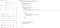Wrong solution! In line 15, when we see that the position has been initialised to true before, we immediately mark as false. However, we need this so that we dont overcount characters. There is hence a problem with this implementation.

The following questions below makes use of Prefix Sum and Hash Map. Good to be able to spot what type of questions needs this.

## Leet Code 974. Subarray Sums Divisible by K

Given an array `A` of integers, return the number of (contiguous, non-empty) subarrays that have a sum divisible by `K`.

Example 1:

`Input: A = [4,5,0,-2,-3,1], K = 5Output: 7Explanation: There are 7 subarrays with a sum divisible by K = 5:[4, 5, 0, -2, -3, 1], , [5, 0], [5, 0, -2, -3], , [0, -2, -3], [-2, -3]`

When we have X%k=2 and Y%k=2, we get (X-Y)%k = 0.

The idea behind this solution is to calculate the cumulative sum of the items in the given array. For eg, at index i we have the sum of the numbers from position 0,1..i. Then for each index, we store the value of cumulative sum % K.

If we have C counts with remainder 2, then total number of subarrays with remainder 0 derived from the subtraction of the cumulative sums with remainder 2 can be visualised as a C Choose 2 problem. We choose any two points and take their difference to give a remainder of 0. (see image above).

The special case is if we have C counts with remainder 0, where the total number of subarrays with remainder 0 will be C + C Choose 2.

Hence, in the code above, we store count (key) and their associated remainder (value) in a hashmap. Formula of C Choose 2 is given to be C*(C-1)/2.

## LeetCode 560. Subarray Sum Equals K

Given an array of integers `nums` and an integer `k`, return the total number of continuous subarrays whose sum equals to `k`.

Example 1:

`Input: nums = [1,1,1], k = 2Output: 2`

Example 2:

`Input: nums = [1,2,3], k = 3Output: 2`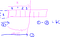We need Line 5 because without it we might miss the subarrays which starts from the first index, and sums up to k.

## Leet Code 1658. Minimum Operations to Reduce X to Zero

You are given an integer array `nums` and an integer `x`. In one operation, you can either remove the leftmost or the rightmost element from the array `nums` and subtract its value from `x`. Note that this modifies the array for future operations.

Return the minimum number of operations to reduce `x` to exactly `0` if it's possible, otherwise, return `-1`.

Example 1:

`Input: nums = [1,1,4,2,3], x = 5Output: 2Explanation: The optimal solution is to remove the last two elements to reduce x to zero.`

Example 2:

`Input: nums = [5,6,7,8,9], x = 4Output: -1`

Example 3:

`Input: nums = [3,2,20,1,1,3], x = 10Output: 5Explanation: The optimal solution is to remove the last three elements and the first two elements (5 operations in total) to reduce x to zero.`

This question is quite similar to the one above involving the use of prefix sum.

• Very important to identify this problem to be like the problem involving prefix sum and map.

## Leet Code 1477. Find Two Non-overlapping Sub-arrays Each With Target Sum

Given an array of integers `arr` and an integer `target`.

You have to find two non-overlapping sub-arrays of `arr` each with sum equal `target`. There can be multiple answers so you have to find an answer where the sum of the lengths of the two sub-arrays is minimum.

Return the minimum sum of the lengths of the two required sub-arrays, or return -1 if you cannot find such two sub-arrays.

Example 1:

`Input: arr = [3,2,2,4,3], target = 3Output: 2Explanation: Only two sub-arrays have sum = 3 ( and ). The sum of their lengths is 2.`

Example 2:

`Input: arr = [7,3,4,7], target = 7Output: 2Explanation: Although we have three non-overlapping sub-arrays of sum = 7 (, [3,4] and ), but we will choose the first and third sub-arrays as the sum of their lengths is 2.`

Example 3:

`Input: arr = [4,3,2,6,2,3,4], target = 6Output: -1Explanation: We have only one sub-array of sum = 6.`

Example 4:

`Input: arr = [5,5,4,4,5], target = 3Output: -1Explanation: We cannot find a sub-array of sum = 3.`

Example 5:

`Input: arr = [3,1,1,1,5,1,2,1], target = 3Output: 3Explanation: Note that sub-arrays [1,2] and [2,1] cannot be an answer because they overlap.`

This problem uses prefix sum and the Transactions Stock (At most k Transaction) idea.

At position i, we visualise as cutting the array into two partition. [0,1…i] and [i+1, i+2…]. For each i, we compute the total length of the subarrays (that sums to target) in these 2 partitions. For each i, we track the minimum length and return that.

`length = MIN {leftIndex[i] + rightIndex[i]} for each i value`

Hence, we need to first use the idea of prefix sum to obtain values at every i position for leftIndex and rightIndex.

rightIndex[i] contains min length of subarray that sums up to target from position rightIndex.length-1, rightIndex.length-2,…i+1.

leftIndex[i] contains min length of subarray that sums up to target from position 0,1…i.

## Leet Code 713. Subarray Product Less Than K

Your are given an array of positive integers `nums`.

Count and print the number of (contiguous) subarrays where the product of all the elements in the subarray is less than `k`.

Example 1:

`Input: nums = [10, 5, 2, 6], k = 100Output: 8Explanation: The 8 subarrays that have product less than 100 are: , , , , [10, 5], [5, 2], [2, 6], [5, 2, 6].Note that [10, 5, 2] is not included as the product of 100 is not strictly less than k.`

This is a Sliding Window Question.

## 1524. Number of Sub-arrays With Odd Sum

Given an array of integers `arr`. Return the number of sub-arrays with odd sum.

As the answer may grow large, the answer must be computed modulo `10^9 + 7`.

Example 1:

`Input: arr = [1,3,5]Output: 4Explanation: All sub-arrays are [,[1,3],[1,3,5],,[3,5],]All sub-arrays sum are [1,4,9,3,8,5].Odd sums are [1,9,3,5] so the answer is 4.`

Example 2:

`Input: arr = [2,4,6]Output: 0Explanation: All sub-arrays are [,[2,4],[2,4,6],,[4,6],]All sub-arrays sum are [2,6,12,4,10,6].All sub-arrays have even sum and the answer is 0.`

Example 3:

`Input: arr = [1,2,3,4,5,6,7]Output: 16`

Example 4:

`Input: arr = [100,100,99,99]Output: 4`

Example 5:

`Input: arr = Output: 1`

ODD — ODD = EVEN

ODD — EVEN = ODD

EVEN — ODD = ODD

EVEN — EVEN = EVEN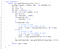The addition of mod is just for the test case when the input has big numbers. Can ignore….

Hackerank Pairs

# Pairs

## LeetCode 5 Longest Palindrome Substring

Given a string `s`, return the longest palindromic substring in `s`.

Example 1:

`Input: s = "babad"Output: "bab"Note: "aba" is also a valid answer.`

Example 2:

`Input: s = "cbbd"Output: "bb"`

## Leet Code 516. Longest Palindromic Subsequence

Given a string s, find the longest palindromic subsequence’s length in s. You may assume that the maximum length of s is 1000.

Example 1:
Input:

`"bbbab"`

Output:

`4`

One possible longest palindromic subsequence is “bbbb”.

Example 2:
Input:

`"cbbd"`

Output:

`2`

One possible longest palindromic subsequence is “bb”.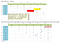When current character matches another character (not itself), we add 2 (itself and it’s match) to the value at position [i+1][j-1], one row below and one column before. This gives the middle substrings (palindrome) between current character and it’s match.

Those unfilled positions are 0.

## Leet Code 9: Palindrome Number

Determine whether an integer is a palindrome. An integer is a palindrome when it reads the same backward as forward.

Follow up: Could you solve it without converting the integer to a string?

Example 1:

`Input: x = 121Output: true`

Example 2:

`Input: x = -121Output: falseExplanation: From left to right, it reads -121. From right to left, it becomes 121-. Therefore it is not a palindrome.`

Example 3:

`Input: x = 10Output: falseExplanation: Reads 01 from right to left. Therefore it is not a palindrome.`

Example 4:

`Input: x = -101Output: false`

The first step is to find out the number of digits that the given input has and determine if it is an odd or even number.

Then, we add the last half of the digit into a number and compare with the first half.

Note that negative values are not palindromes.

## Leet Code Solution:

Reversing number: For number `1221`, if we do `1221 % 10`, we get the last digit `1`, to get the second to the last digit, we need to remove the last digit from `1221`, we could do so by dividing it by 10, `1221 / 10 = 122`. Then we can get the last digit again by doing a modulus by 10, `122 % 10 = 2`, and if we multiply the last digit by 10 and add the second last digit, `1 * 10 + 2 = 12`, it gives us the reverted number we want. Continuing this process would give us the reverted number with more digits.

Now the question is, how do we know that we’ve reached the half of the number?

Since we divided the number by 10, and multiplied the reversed number by 10, when the original number is less than the reversed number, it means we’ve processed half of the number digits.

## Leet Code 336. Palindrome Pairs (Hard)

Given a list of unique words, return all the pairs of the distinct indices `(i, j)` in the given list, so that the concatenation of the two words `words[i] + words[j]` is a palindrome.

Example 1:

`Input: words = ["abcd","dcba","lls","s","sssll"]Output: [[0,1],[1,0],[3,2],[2,4]]Explanation: The palindromes are ["dcbaabcd","abcddcba","slls","llssssll"]`

Example 2:

`Input: words = ["bat","tab","cat"]Output: [[0,1],[1,0]]Explanation: The palindromes are ["battab","tabbat"]`

Example 3:

`Input: words = ["a",""]Output: [[0,1],[1,0]]`

`TODO`

## 131. Palindrome Partitioning

Given a string `s`, partition `s` such that every substring of the partition is a palindrome. Return all possible palindrome partitioning of `s`.

A palindrome string is a string that reads the same backward as forward.

Example 1:

`Input: s = "aab"Output: [["a","a","b"],["aa","b"]]`

Example 2:

`Input: s = "a"Output: [["a"]]`

The param index is the starting index.

O(n) complexity in each recursion call.

Max recursion depth is n — length of string. O(n) calls each.

Total complexity: O(n*n)

How can we improve?

We can further optimize the approach by using dynamic programming to determine if a string is a palindrome in constant time.

We use a 2 Dimensional array dp of size NN where,

dp[start][end] = true , if the substring beginning at index start and ending at index end is a palindrome.

Otherwise, dp[start][end] == false.

Also, we must update the dp array, if we find that the current string is a palindrome.

`TODO`

## Increasing Triplet Subsequence

Given an unsorted array return whether an increasing subsequence of length 3 exists or not in the array.

Formally the function should:

Return true if there exists i, j, k
such that
arr[i] < arr[j] < arr[k] given 0 ≤ i < j < kn-1 else return false.

Note: Your algorithm should run in O(n) time complexity and O(1) space complexity.

Example 1:

`Input: [1,2,3,4,5]Output: true`

Example 2:

`Input: [5,4,3,2,1]Output: false`
• If the current number is bigger than the secondSmallest, we know for sure that we have seen 3 increasing subsequence hence we can immediately return true.
• If the current number is between smallest and secondSmallest, then we need to update secondSmallest since that is no longer valid.
• If current number is smaller than smallest variable, then it means current smallest value is no longer valid. Hence we need to update it. We do not need to update secondSmallest because we know for sure that the current number is smaller than secondSmallest variable since we passed the first if.
• We do not update secondSmallest to hold the value of the previous smallest value (the one we just updated) because the ordering should be that smallest variable has lower index than secondSmallest. In this case, if we update as seen in line 17, the index of the number in the secondSmallest variable is smaller than that in smallest variable, which is wrong. We want secondSmallest variable to hold the value with a greater index than the value in smallest variable.
• At this point, one question that comes to mind is that when in the third if loop if we only update the variable smallest, then wouldnt the ordering be wrong as well. Since we updated secondSmallest before we updated smallest variable, this means the index of secondSmallest value is smaller than index of smallest value. Then, you might try to do the screenshot below.
• But it doesnt work as well. It fails the test case here:
• The reason why it fails is because when we reach the -1, we update smallest = -1, secondSmallest = Integer.MAX_VALUE. Then we lost the [0,2,3] increasing subsequence since when we reach 3, we see that 3 is smaller than secondSmallest variable which holds max value and then we wrongly return False. In this case, perhaps the ordering does not matter much. As long as we know for sure that the secondSmallest variable contains a value that is bigger than at least 1 value that comes before it, we don’t have to update it. When we update the smallest variable in the 3rd If, we are simply making the smallest variable smaller but the two variables no longer contain the values of the increasing subsequence.

## Letter Combinations of a phone number

Given a string containing digits from `2-9` inclusive, return all possible letter combinations that the number could represent. Return the answer in any order.

A mapping of digit to letters (just like on the telephone buttons) is given below. Note that 1 does not map to any letters.

Example 1:

`Input: digits = "23"Output: ["ad","ae","af","bd","be","bf","cd","ce","cf"]`

Example 2:

`Input: digits = ""Output: []`

Example 3:

`Input: digits = "2"Output: ["a","b","c"]`

The idea is to make use of recursion to keep trying out all possible combinations of strings. The variable `position `indicate which index of the input string `digits` we are currently at. Each iteration is concerned with appending the given String variable `curr` , accumulated from previous iterations with a single character String. This single character String is the possible value that the `position` might represent (as we initialised in the HashMap).

When we have tried out all possible combinations, we will add the final string into the global list.

## Leet Code 153. Find Minimum in Rotated Sorted Array

Suppose an array of length `n` sorted in ascending order is rotated between `1` and `n` times. For example, the array `nums = [0,1,2,4,5,6,7]` might become:

• `[4,5,6,7,0,1,2]` if it was rotated `4` times.
• `[0,1,2,4,5,6,7]` if it was rotated `7` times.

Notice that rotating an array `[a, a, a, ..., a[n-1]]` 1 time results in the array `[a[n-1], a, a, a, ..., a[n-2]]`.

Given the sorted rotated array `nums`, return the minimum element of this array.

Example 1:

`Input: nums = [3,4,5,1,2]Output: 1Explanation: The original array was [1,2,3,4,5] rotated 3 times.`

Example 2:

`Input: nums = [4,5,6,7,0,1,2]Output: 0Explanation: The original array was [0,1,2,4,5,6,7] and it was rotated 4 times.`

Example 3:

`Input: nums = [11,13,15,17]Output: 11Explanation: The original array was [11,13,15,17] and it was rotated 4 times.`

The big idea is to look at the pattern and figure out where exactly could the smallest value be located, when we are at the mid point of the array.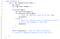Note the while condition -> Because when low=high, it means we are at the minimum value. If we have the condition to be low≤high, then it will lead to infinity loop.

## Leet Code 154. Find Minimum in Rotated Sorted Array II

Suppose an array sorted in ascending order is rotated at some pivot unknown to you beforehand.

(i.e., `[0,1,2,4,5,6,7]` might become `[4,5,6,7,0,1,2]`).

Find the minimum element.

The array may contain duplicates.

Example 1:

`Input: [1,3,5]Output: 1`

Example 2:

`Input: [2,2,2,0,1]Output: 0`

The only change would be from line 11 to 15.

If we satisfy the first IF condition at line 8, it must mean that the minimum value is to the RIGHT of num[mid]. The items to the RHS are sorted in increasing order (optional) then decreasing and then possibly increasing (optional).

If we do not satisfy the first IF condition, it means nums[mid]≤nums[high]. This means the items to the RHS is definitely INCREASING. The minimum condition will be on the LEFT of num[mid].

Second IF condition is If we see that the value at mid is smaller than that in low. Then, it means LEFT side is increasing (optional) then decreasing. Minimum value is definitely on left.

Third IF condition is for when there are duplicates. When the left side values are all the same. Then we will pass the second IF condition as well and we know this repeated value is then the minimum. So we just decrement high by 1.

## Leet Code 33. Search in Rotated Sorted Array

You are given an integer array `nums` sorted in ascending order, and an integer `target`.

Suppose that `nums` is rotated at some pivot unknown to you beforehand (i.e., `[0,1,2,4,5,6,7]` might become `[4,5,6,7,0,1,2]`).

If `target` is found in the array return its index, otherwise, return `-1`.

Example 1:

`Input: nums = [4,5,6,7,0,1,2], target = 0Output: 4`

Example 2:

`Input: nums = [4,5,6,7,0,1,2], target = 3Output: -1`

Example 3:

`Input: nums = , target = 0Output: -1`

## Leet Code 4. Median of Two Sorted Arrays

Given two sorted arrays `nums1` and `nums2` of size `m` and `n` respectively, return the median of the two sorted arrays.

Follow up: The overall run time complexity should be `O(log (m+n))`.

Example 1:

`Input: nums1 = [1,3], nums2 = Output: 2.00000Explanation: merged array = [1,2,3] and median is 2.`

Example 2:

`Input: nums1 = [1,2], nums2 = [3,4]Output: 2.50000Explanation: merged array = [1,2,3,4] and median is (2 + 3) / 2 = 2.5.`

Example 3:

`Input: nums1 = [0,0], nums2 = [0,0]Output: 0.00000`

Example 4:

`Input: nums1 = [], nums2 = Output: 1.00000`

Example 5:

`Input: nums1 = , nums2 = []Output: 2.00000`

This question is hard…

• We want nums1 to contain the shorter array and nums2 to contain the longer array.
• We then do modified binary search on this smaller array.
• cut1 variable contains the number of elements in nums1 to the left of the cut made on num1 array. The first time we do, (0+len1)/2. In the array with 5 elements, it will be (0+5)/2 = 2.5 = 2. The first cut is after the second element at index 1.
• cut2 variable contains the number of elements in nums2 to the left of the cut made on num2 array. We calculate this from cut1 variable. Note that we want the cut to be at the median position. Median should be at half the length of the total len of the two arrays combined. For eg if we calculate median to be of length X, then since we previously calculated num1 to be contributing cut1 number to the left side of this median point, then num2 will contribute X-cut1 number of elements to the left side of this median point. Hence, we have X-num1 where X=(len+1)/2. The reason why it is (len+1) is so that if the total length of array is odd number, we want the left side of median to contain 1 more element than right side of the median.
• cut1==0 // no elements on left
• cut1==len1 // no elements on right
• With the cut determined, we then make sure that the condition holds `l1<r2 and l2<r1`
• If this condition is violated (detected by the IF ELSE loops), then we need to modify the binary search low and high values in num1 array.
• If this condition is not violated, then we have reached the end and can return the median value.

## Leet Code 7 Reverse Integer

Given a 32-bit signed integer, reverse digits of an integer.

Note:
Assume we are dealing with an environment that could only store integers within the 32-bit signed integer range: [−231, 231 − 1]. For the purpose of this problem, assume that your function returns 0 when the reversed integer overflows.

Example 1:

`Input: x = 123Output: 321`

Example 2:

`Input: x = -123Output: -321`

Example 3:

`Input: x = 120Output: 21`

Example 4:

`Input: x = 0Output: 0`

## Leet Code 88. Merge Sorted Array

Given two sorted integer arrays nums1 and nums2, merge nums2 into nums1 as one sorted array.

Note:

• The number of elements initialized in nums1 and nums2 are m and n respectively.
• You may assume that nums1 has enough space (size that is equal to m + n) to hold additional elements from nums2.

Example:

`Input:nums1 = [1,2,3,0,0,0], m = 3nums2 = [2,5,6],       n = 3Output: [1,2,2,3,5,6]`

## Leet Code 30. Substring with Concatenation of All Words

You are given a string `s` and an array of strings `words` of the same length. Return all starting indices of substring(s) in `s` that is a concatenation of each word in `words` exactly once, in any order, and without any intervening characters.

You can return the answer in any order.

Example 1:

`Input: s = "barfoothefoobarman", words = ["foo","bar"]Output: [0,9]Explanation: Substrings starting at index 0 and 9 are "barfoo" and "foobar" respectively.The output order does not matter, returning [9,0] is fine too.`

Example 2:

`Input: s = "wordgoodgoodgoodbestword", words = ["word","good","best","word"]Output: []`

Example 3:

`Input: s = "barfoofoobarthefoobarman", words = ["bar","foo","the"]Output: [6,9,12]`

## Leet Code 31. Next Permutation

Implement next permutation, which rearranges numbers into the lexicographically next greater permutation of numbers.

If such an arrangement is not possible, it must rearrange it as the lowest possible order (i.e., sorted in ascending order).

The replacement must be in place and use only constant extra memory.

Example 1:

`Input: nums = [1,2,3]Output: [1,3,2]`

Example 2:

`Input: nums = [3,2,1]Output: [1,2,3]`

Example 3:

`Input: nums = [1,1,5]Output: [1,5,1]`

Example 4:

`Input: nums = Output: `

This question is quite hard. Need to spot the pattern.

Leet code given solution:

First, we observe that a sequence in descending order has no next larger permutation.

We need to find the first pair of two successive numbers a[i]and a[i-1], from the right, which satisfy a[i-1] < a[i]. For eg, `36XXX where 6 is larger than 3.` Now, no rearrangements to the right of a[i-1] (section XXX)can create a larger permutation since that subarray consists of numbers in descending order.

We want to create the permutation just larger than the current one. Therefore, we need to replace the number a[i-1] with the number which is just larger than itself among the numbers lying to its right section, say a[j], one of x.

We swap the numbers a[i-1] and a[j]. We now have the correct number at index i-1. But still the current permutation isn’t the permutation that we are looking for. We need the smallest permutation that can be formed by using the numbers only to the right of a[i-1]. Therefore, we need to place those numbers in ascending order to get their smallest permutation. Therefore, we simply need to reverse the numbers following a[i-1] to get the next smallest lexicographic permutation.

## Leet Code 35. Search Insert Position

Given a sorted array of distinct integers and a target value, return the index if the target is found. If not, return the index where it would be if it were inserted in order.

Example 1:

`Input: nums = [1,3,5,6], target = 5Output: 2`

Example 2:

`Input: nums = [1,3,5,6], target = 2Output: 1`

Example 3:

`Input: nums = [1,3,5,6], target = 7Output: 4`

Example 4:

`Input: nums = [1,3,5,6], target = 0Output: 0`

Example 5:

`Input: nums = , target = 0Output: 0`

## Leet Code 36 Valid Sudoku

Determine if a `9 x 9` Sudoku board is valid. Only the filled cells need to be validated according to the following rules:

1. Each row must contain the digits `1-9` without repetition.
2. Each column must contain the digits `1-9` without repetition.
3. Each of the nine `3 x 3` sub-boxes of the grid must contain the digits `1-9` without repetition.

Note:

• A Sudoku board (partially filled) could be valid but is not necessarily solvable.
• Only the filled cells need to be validated according to the mentioned rules.

Leet Code Solution:

One could use `box_index = (row / 3) * 3 + col / 3` where `/` is an integer division, `row` is a row number, and `col` is a column number.

## 41. First Missing Positive (Hard)

Given an unsorted integer array `nums`, find the smallest missing positive integer.

Follow up: Could you implement an algorithm that runs in `O(n)` time and uses constant extra space.?

Example 1:

`Input: nums = [1,2,0]Output: 3`

Example 2:

`Input: nums = [3,4,-1,1]Output: 2`

Example 3:

`Input: nums = [7,8,9,11,12]Output: 1`

## 218. The Skyline Problem

A city’s skyline is the outer contour of the silhouette formed by all the buildings in that city when viewed from a distance. Given the locations and heights of all the buildings, return the skyline formed by these buildings collectively.

The geometric information of each building is given in the array `buildings` where `buildings[i] = [lefti, righti, heighti]`:

• `lefti` is the x coordinate of the left edge of the `ith` building.
• `righti` is the x coordinate of the right edge of the `ith` building.
• `heighti` is the height of the `ith` building.

You may assume all buildings are perfect rectangles grounded on an absolutely flat surface at height `0`.

The skyline should be represented as a list of “key points” sorted by their x-coordinate in the form `[[x1,y1],[x2,y2],...]`. Each key point is the left endpoint of some horizontal segment in the skyline except the last point in the list, which always has a y-coordinate `0` and is used to mark the skyline's termination where the rightmost building ends. Any ground between the leftmost and rightmost buildings should be part of the skyline's contour.

Note: There must be no consecutive horizontal lines of equal height in the output skyline. For instance, `[...,[2 3],[4 5],[7 5],[11 5],[12 7],...]` is not acceptable; the three lines of height 5 should be merged into one in the final output as such: `[...,[2 3],[4 5],[12 7],...]`

Example 1:

`Input: buildings = [[2,9,10],[3,7,15],[5,12,12],[15,20,10],[19,24,8]]Output: [[2,10],[3,15],[7,12],[12,0],[15,10],[20,8],[24,0]]Explanation:Figure A shows the buildings of the input.Figure B shows the skyline formed by those buildings. The red points in figure B represent the key points in the output list.`

Example 2:

`Input: buildings = [[0,2,3],[2,5,3]]Output: [[0,3],[5,0]]`

Leet Code Solution:

Copied from a YouTube tutorial on this question.

The idea is to maintain 2 data structures: (1) TreeMap where the key indicates the X-Coord, Value is an ArrayList of int array of values (start, end, height). This array list of int arrays are buildings that start with the key X Coord. (2) Max Heap which contains the height of all the buildings. As we iterate through the Tree Map, we will update this Max Heap. At any point, the max heap will contain the heights of all the buildings presently at the key value (X-Coord) we are iterating at in the Tree Map.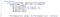For each building, we add the and to the Tree Map.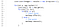a: current X-Coord we are at. For the value a we are at, we have a list of buildings located at a position. We then proceed to check for each of these buildings, whether it is their starting or ending point at a position. If it is starting position of a building at a, then we add to max heap. Otherwise, we remove from max heap since we always want max heap to be updated and to contain all the buildings that are present in the current a position we are iterating at, in the Tree Map.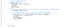Then, we check the size of Max Heap. IF size is 0, it means there are no more buildings at the current position a. So we need to add to results to indicate the key point of having height 0. OTHERWISE, we then check the height of the last entry of the results list. If that height is different from the max height in the max heap, it means at the current position, there is a new height, hence key point to be added.

## Leet Code 295. Find Median from Data Stream

Median is the middle value in an ordered integer list. If the size of the list is even, there is no middle value. So the median is the mean of the two middle value.

For example,

`[2,3,4]`, the median is `3`

`[2,3]`, the median is `(2 + 3) / 2 = 2.5`

Design a data structure that supports the following two operations:

• void addNum(int num) — Add a integer number from the data stream to the data structure.
• double findMedian() — Return the median of all elements so far

## Leet Code 249. Group Shifted Strings (Medium)

Given a string, we can “shift” each of its letter to its successive letter, for example: `"abc" -> "bcd"`. We can keep "shifting" which forms the sequence:

`"abc" -> "bcd" -> ... -> "xyz"`

Given a list of strings which contains only lowercase alphabets, group all strings that belong to the same shifting sequence.

For example, given: `["abc", "bcd", "acef", "xyz", "az", "ba", "a", "z"]`,
A solution is:

`[  ["abc","bcd","xyz"],  ["az","ba"],  ["acef"],  ["a","z"]]`

Two strings are considered to be in the same group if we can shift those strings and get to the same string. The idea to solve this question is to compute the difference between each character in a string, then store them in a stack. For eg, if i have a word “abe” then the stack will contain [1,3]. We have a global hash map where the key would be the stack of integers and the values would be a list of string that has this key stack.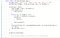Line 16 could be s.charAt(i) — s.charAt(i-1). Although this may work too.

Note that we have to check if the difference is smaller than 0 and add 26 to it. This is because we need to account for wrapping…

## Leet Code 76. Minimum Window Substring (Hard)

Given two strings `s` and `t`, return the minimum window in `s` which will contain all the characters in `t`. If there is no such window in `s` that covers all characters in `t`, return the empty string `""`.

Note that If there is such a window, it is guaranteed that there will always be only one unique minimum window in `s`.

Example 1:

`Input: s = "ADOBECODEBANC", t = "ABC"Output: "BANC"`

Example 2:

`Input: s = "a", t = "a"Output: "a"`

This is the sliding window method.

## 345. Reverse Vowels of a String

Write a function that takes a string as input and reverse only the vowels of a string.

Example 1:

`Input: "hello"Output: "holle"`

Example 2:

`Input: "leetcode"Output: "leotcede"`

## Leet Code 829. Consecutive Numbers Sum

Given a positive integer `N`, how many ways can we write it as a sum of consecutive positive integers?

Example 1:

`Input: 5Output: 2Explanation: 5 = 5 = 2 + 3`

Example 2:

`Input: 9Output: 3Explanation: 9 = 9 = 4 + 5 = 2 + 3 + 4`

Example 3:

`Input: 15Output: 4Explanation: 15 = 15 = 8 + 7 = 4 + 5 + 6 = 1 + 2 + 3 + 4 + 5`

Using prefix sum method works for some of the test cases but fails for others when the number gets big. This is due to Integer overflow. Nevertheless, i will still put my attempt.

The correct Solution: Too complicated to understand, seriously….`TODO`

## 151. Reverse Words in a String

Given an input string `s`, reverse the order of the words.

A word is defined as a sequence of non-space characters. The words in `s` will be separated by at least one space.

Return a string of the words in reverse order concatenated by a single space.

Note that `s` may contain leading or trailing spaces or multiple spaces between two words. The returned string should only have a single space separating the words. Do not include any extra spaces.

Example 1:

`Input: s = "the sky is blue"Output: "blue is sky the"`

Example 2:

`Input: s = "  hello world  "Output: "world hello"Explanation: Your reversed string should not contain leading or trailing spaces.`

Example 3:

`Input: s = "a good   example"Output: "example good a"Explanation: You need to reduce multiple spaces between two words to a single space in the reversed string.`

Example 4:

`Input: s = "  Bob    Loves  Alice   "Output: "Alice Loves Bob"`

Example 5:

`Input: s = "Alice does not even like bob"Output: "bob like even not does Alice"`

The idea is to reverse the given string. Then, for each word segment separated by space, we reverse the word.

Since the given string might have spacing (extra) between words or outside of the sentence, we need to remove them.

## 209. Minimum Size Subarray Sum

Given an array of n positive integers and a positive integer s, find the minimal length of a contiguous subarray of which the sum ≥ s. If there isn’t one, return 0 instead.

Example:

`Input: s = 7, nums = [2,3,1,2,4,3]Output: 2Explanation: the subarray [4,3] has the minimal length under the problem constraint.`

Sort of like sliding window.

The thing to note is line 18. We compute the length to be end — start instead of (end-start+1), this is because the end index would be incremented by 1 and then it fails the while condition. This means the end index is not valid/not included in making the sum.

## 1679. Max Number of K-Sum Pairs

You are given an integer array `nums` and an integer `k`.

In one operation, you can pick two numbers from the array whose sum equals `k` and remove them from the array.

Return the maximum number of operations you can perform on the array.

Example 1:

`Input: nums = [1,2,3,4], k = 5Output: 2Explanation: Starting with nums = [1,2,3,4]:- Remove numbers 1 and 4, then nums = [2,3]- Remove numbers 2 and 3, then nums = []There are no more pairs that sum up to 5, hence a total of 2 operations.`

Example 2:

`Input: nums = [3,1,3,4,3], k = 6Output: 1Explanation: Starting with nums = [3,1,3,4,3]:- Remove the first two 3's, then nums = [1,4,3]There are no more pairs that sum up to 6, hence a total of 1 operation.`

## 348. Design Tic-Tac-Toe

Design a Tic-tac-toe game that is played between two players on anxngrid.

You may assume the following rules:

1. A move is guaranteed to be valid and is placed on an empty block.
2. Once a winning condition is reached, no more moves is allowed.
3. A player who succeeds in placing n of their marks in a horizontal, vertical, or diagonal row wins the game.

The O(n²) algorithm is pretty easy to derive….but can we do better?

## 1498. Number of Subsequences That Satisfy the Given Sum Condition

Given an array of integers `nums` and an integer `target`.

Return the number of non-empty subsequences of `nums` such that the sum of the minimum and maximum element on it is less or equal to `target`. Since the answer may be too large, return it modulo `109 + 7`.

Example 1:

`Input: nums = [3,5,6,7], target = 9Output: 4Explanation: There are 4 subsequences that satisfy the condition. -> Min value + max value <= target (3 + 3 <= 9)[3,5] -> (3 + 5 <= 9)[3,5,6] -> (3 + 6 <= 9)[3,6] -> (3 + 6 <= 9)`

Example 2:

`Input: nums = [3,3,6,8], target = 10Output: 6Explanation: There are 6 subsequences that satisfy the condition. (nums can have repeated numbers). ,  , [3,3], [3,6] , [3,6] , [3,3,6]`

Example 3:

`Input: nums = [2,3,3,4,6,7], target = 12Output: 61Explanation: There are 63 non-empty subsequences, two of them don't satisfy the condition ([6,7], ).Number of valid subsequences (63 - 2 = 61).`

Example 4:

`Input: nums = [5,2,4,1,7,6,8], target = 16Output: 127Explanation: All non-empty subset satisfy the condition (2^7 - 1) = 127`

Check out this useful youtube explanation.

The idea is to first sort the array.

You may ask, doesn’t the ordering matter? The answer is, no. If you sort it, the only thing that is different is the ordering of the subsequences but essentially the set of values in an identified subsequence is the same. See the above example.

Once the array has been sorted, we will then proceed to compute.

We will iterate through every element in the array. We visualise the problem to be fixing the element the pointer is at, called X, to be a part of the subsequence we are going to form. Ignore all numbers on the left of it as they have been processed already. Then, for the numbers on the right, as long as we encounter another number Y whereby X+Y≤target, we will increment a counter (initialised to 0 at every iteration). Stop when the condition does not hold. Eventually, we will add 2^counter to the result. See the picture below.

At index 0, there are two numbers 3 and 6 which satisfy the condition. At index 1, there is one number 6 which satisfy the condition. At index 2, no number on the RHS satisfy the condition. Hence, result is 6.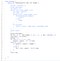Not sure why my attempt (commented) is wrong..below was taken from leetcode discussion

# Smallest Substring of All Characters

## 1052. Grumpy Bookstore Owner

Today, the bookstore owner has a store open for `customers.length` minutes. Every minute, some number of customers (`customers[i]`) enter the store, and all those customers leave after the end of that minute.

On some minutes, the bookstore owner is grumpy. If the bookstore owner is grumpy on the i-th minute, `grumpy[i] = 1`, otherwise `grumpy[i] = 0`. When the bookstore owner is grumpy, the customers of that minute are not satisfied, otherwise they are satisfied.

The bookstore owner knows a secret technique to keep themselves not grumpy for `X` minutes straight, but can only use it once.

Return the maximum number of customers that can be satisfied throughout the day.

Example 1:

`Input: customers = [1,0,1,2,1,1,7,5], grumpy = [0,1,0,1,0,1,0,1], X = 3Output: 16Explanation: The bookstore owner keeps themselves not grumpy for the last 3 minutes. The maximum number of customers that can be satisfied = 1 + 1 + 1 + 1 + 7 + 5 = 16.`

# The End for now :)

This is a repository of my thoughts on my personal life, my random interests & notes taken down as I navigate my way through the tech world!

## More from LiveRunGrow

This is a repository of my thoughts on my personal life, my random interests & notes taken down as I navigate my way through the tech world!